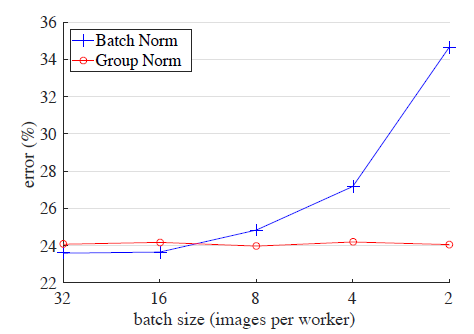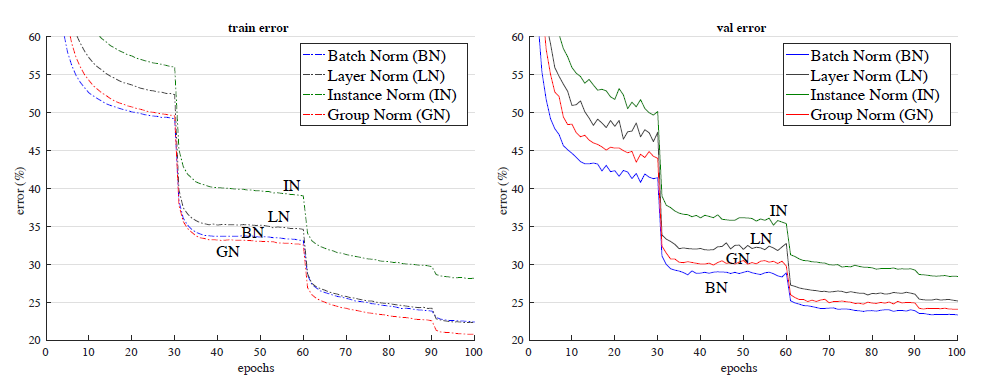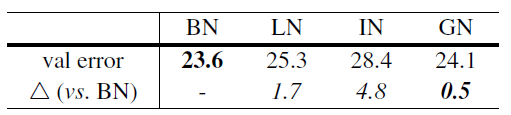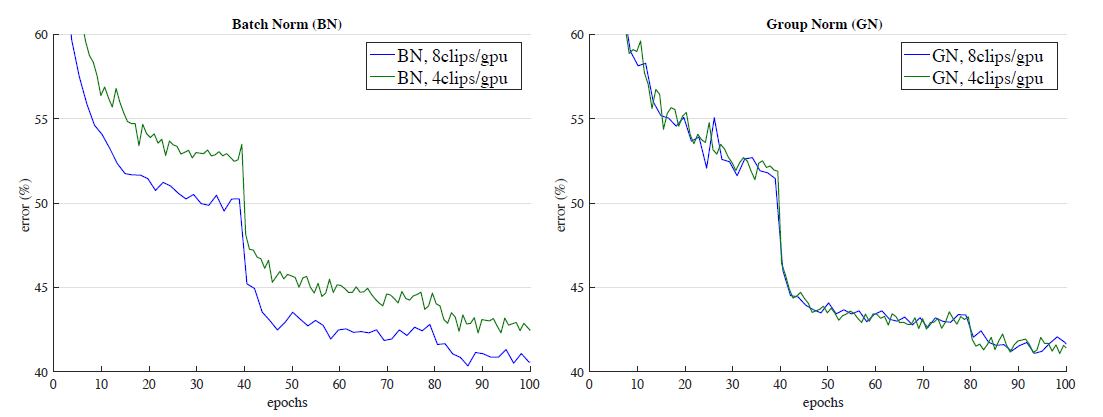# Review — Group Norm (GN): Group Normalization (Image Classification)

## Suitable for Memory-Constraint Applications With Small Batch Size Like Object Detection & Segmentation, Outperforms Batch Norm (BN), Layer Norm (LN) & Instance Norm (IN)Error Rate by Batch Norm (BN) Increases When Batch Size Decreasing While Group Norm (GN) Still Can Maintain Similar Error Rate

In this story, Group Normalization, Group Norm (GN), by Facebook AI Research (FAIR), is presented.

In conventional Batch Norm (BN):

• Normalizing along the batch dimension introduces problems — BN error increases rapidly when the batch size becomes smaller, caused by inaccurate batch statistics estimation.
• This limits BN’s usage for training larger models, like computer vision tasks including detection, segmentation, and video.

In this paper:

• Group Normalization (GN) is treated as a simple alternative to BN.
• GN divides the channels into groups and computes within each group the mean and variance for normalization.
• GN’s computation is independent of batch sizes, and its accuracy is stable in a wide range of batch sizes.

This is a paper in 2018 ECCV with over 900 citations. (Sik-Ho Tsang @ Medium)

# Outline

1. Normalization Methods: BN, LN, IN
2. Group Normalization (GN)
3. Image Classification Results in ImageNet
4. Object Detection and Segmentation in COCO

# 1. Normalization Methods: BN, LN, IN

• In Batch Norm (BN), Layer Norm (LN), Instance Norm (IN), and Group Norm (GN):
• where xi is the feature, and ^xi is the feature normalized by μ and σ where μ and σ are the mean and standard deviation (std):
• where Si is the set of pixels in which the mean and std are computed, and m is the size of this set.

Depending on the norm type, μ and σ can be calculated along N (batch axis), C (channel axis), H (spatial height axis) and W (spatial width axis).

• In Layer Norm (LN), LN computes μ and σ along the (C, H, W) axes.
• where Layer Norm was originally proposed for recurrent neural network (RNN) by Hinton’s research group.
• In Instance Norm (IN), IN computes μ and σ along the (H, W) axes for each sample and each channel.
• where Instance Norm was originally proposed for texture stylization.
• All methods of BN, LN, and IN learn a per-channel linear transform to compensate for the possible lost of representational ability:
• where γ and β are trainable scale and shift.

# 2. Group Normalization (GN)

• Formally, a Group Norm layer computes μ and σ in a set Si defined as:
• Here G is the number of groups, which is a pre-defined hyper-parameter (G = 32 by default). C/G is the number of channels per group.
• GN computes μ and σ along the (H,W) axes and along a group of C/G channels.
• In the above figure (rightmost), it is a simple case of 2 groups (G = 2) each having 3 channels.
• Specifically, the pixels in the same group are normalized together by the same μ and σ. GN also learns the per-channel γ and β.
• GN can be easily implemented by a few lines of code in either Pytorch and TensorFlow, as shown above.

## 2.1. Relation to Layer Normalization

• GN becomes LN if we set the group number as G = 1. GN is less restricted than LN, because each group of channels (instead of all of them) are assumed to subject to the shared mean and variance.
• The model still has flexibility of learning a different distribution for each group. This leads to improved representational power of GN over LN.

## 2.2. Relation to Instance Normalization

• GN becomes IN if we set the group number as G = C (i.e., one channel per group). But IN can only rely on the spatial dimension for computing the mean and variance and it misses the opportunity of exploiting the channel dependence.

# 3. Image Classification Results in ImageNet

## 3.1. Regular Batch SizeComparison of error curves with a batch size of 32 images/GPUComparison of error rates (%) of ResNet-50 in the ImageNet validation set, trained with a batch size of 32 images/GPU
• In this regime where BN works well, GN is able to approach BN’s accuracy, with a decent degradation of 0.5% in the validation set.
• Actually, the above figure (left) shows that GN has lower training error than BN, indicating that GN is effective for easing optimization.
• The slightly higher validation error of GN implies that GN loses some regularization ability of BN because BN’s mean and variance computation introduces uncertainty caused by the stochastic batch sampling, which helps regularization.

## 3.2. Small Batch Size

• Although BN benefits from the stochasticity under some situations, its error increases when the batch size becomes smaller and the uncertainty gets bigger, as shown above.
• GN has very similar curves (subject to random variations) across a wide range of batch sizes from 32 to 2.
• In the case of a batch size of 2, GN has 10.6% lower error rate than its BN counterpart (24.1% vs. 34.7%).

## 3.3. Group Division

• GN performs reasonably well for all values of G.
• In the extreme case of G = 1, GN is equivalent to LN.
• In the extreme case of 1 channel per group, GN is equivalent to IN.

## 3.4 Results and Analysis of VGG Models

• The above figure shows the evolution of the feature distributions of conv5_3 (the last convolutional layer).
• GN and BN behave qualitatively similar. This comparison suggests that performing normalization is essential for controlling the distribution of features.

# 4. Object Detection and Segmentation in COCO

• Evaluation is also performed by fine-tuning the models for transferring to object detection and segmentation.

## 4.1. Results of C4 Backbone

• This C4 variant uses ResNet’s layers of up to conv4 to extract feature maps, and ResNet’s conv5 layers as the Region-of-Interest (RoI) heads for classification and regression.
• On this baseline, GN improves over BN* by 1.1 box AP and 0.8 mask AP. (BN* is the BN without fine-tuning. With fine-tuning, BN result is worse.)
• BN* creates inconsistency between pre-training and fine-tuning (frozen).

## 4.2. Results of FPN Backbone

• By adding GN to all convolutional layers of the box head, the box AP increases by 0.9 to 39.5. This ablation shows that a substantial portion of GN’s improvement for detection is from normalization in the head.
• (Applying BN to the box head is tried but with 9 AP worse.)
• Applying GN to the backbone alone contributes a 0.5 AP gain (from 39.5 to 40.0), suggesting that GN helps when transferring features.
• The full results of GN are shown above. GN increases over BN* by a healthy margin.
• It is found that GN is not fully trained with the default schedule. By training with more iterations (long), the final ResNet-50 GN model (“long”) is 2.2 points box AP and 1.6 points mask AP better than its BN* variant.

## 4.3. Training Mask R-CNN from Scratch

GN allows us to easily investigate training object detectors from scratch (without any pre-training).

• To the best of authors’ knowledge, the numbers (41.0 box AP and 36.4 mask AP) are the best from-scratch results in COCO reported to date.
• They can even compete with the ImageNet-pretrained results in the above table at Section 4.2.

## 4.4. Video Classification in Kinetics

• ResNet-50 Inflated 3D (I3D) convolutional networks are used.
• The models are pre-trained from ImageNet. For both BN and GN, we extend the normalization from over (H, W) to over (T, H, W). The model is fully convolutional in spacetime.
• For the batch size of 8, GN is slightly worse than BN by 0.3% top-1 accuracy and 0.1% top-5. GN is competitive with BN when BN works well.
• For the smaller batch size of 4, GN’s accuracy is kept similar (72.8 / 90.6 vs. 73.0 / 90.6), but is better than BN’s 72.1 / 90.0.
• BN’s accuracy is decreased by 1.2% when the batch size decreases from 8 to 4.

GN helps the model benefit from temporal length, and the longer clip boosts the top-1 accuracy by 1.7% (top-5 1.1%) with the same batch size.Error curves in Kinetics with an input length of 32 frames
• BN’s error curves (left) have a noticeable gap when the batch size decreases from 8 to 4, while GN’s error curves (right) are very similar.

--

--